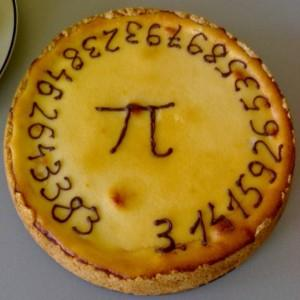* 题图来自 维基百科

•与伪随机

《心理统计课》第二节的操作练习：的十进制表达中，统计 0~9 出现的频率，直至小数点后一百万位（R 代码）。这个例子想解释：主流统计课程（频率学派）的「概率」，可以不牵扯任何随机性。如果造化用的第 10^10^10 位小数开始的数列作为伪随机数发生器，人类只知道这个确定而未知的无穷序列中，0~9 每个数出现的频率收敛到10%（注解见评论）。实际上，几乎所有计算机的（伪）随机数发生器都用某种确定的算法；心理实验设计中的「随机抽样」标准做法也是用确定而未知的伪随机序列，尽可能避免过多的随意性。

• 浮生若• 从「宿命无助」到「使命必达」

Rotter 谦虚地说只做了一点微小的工作，他的原话是「不小心扔了个烟头，整出一场森林大火」——公开发表后的半个世纪，不知已经有多少篇文献采用了他的 I-E量表。这些文献的研究结论绝大多数都符合预期——典型的外控型个体有更强的无助感、更低的成就动机、缺少改变现状的意愿。然而，宗教心理学领域的研究发现了意料之外的反例：如果外控型个体对「无上的神意」具有强烈信仰，反而会表现出内控型个体的典型优点 (Welton, Adkins, Ingle, & Dixon, 1996)。这种伴随「天命自任」体验的决定论带来的是深刻的使命感。《圣经 · 使徒行传》说：「最重要的是完成我的使命，就是主耶稣交给我的工作」，儒家的《孟子》说：「天将降大任于是人」，与宿命只一字之差，使命的决定论世界观足以派生极为积极的人生自我。

• R 代码：来自 π 和 e 小数点后一百万位伪随机数的分布图示
e.m <- read.delim('http://apod.nasa.gov/htmltest/gifcity/e.1mil',header = F)
## from 24 to 12524
as.character(e.m[21:25,1])
as.character(e.m[12521:12525,1])
## string to digits
i= 24:12524
str <- as.character(e.m[i,1])
e.char <- unlist(strsplit(str,''))
table(e.char)
cbind(e.char[1:10]) # " " & "."
e.char <- e.char[ c(-1,-2,-4) ]
table(e.char)
length(e.char)
e.char <- e.char[1: (1+ 1e6)]

pi.char <- unlist(strsplit(pi.m,''))
table(pi.char)
cbind(pi.char[1:10])  # "."
pi.char <- pi.char[-2]
table(pi.char);length(pi.char)

e_pi <- data.frame(e.char,pi.char)
# write.csv(e_pi,file = file.choose(),row.names = F)

layout(cbind(1:2))
plot(as.factor(e.char),main='10^6 digits', sub='e');
plot(as.factor(pi.char),main='10^6 digits', sub=expression(pi));
layout(1)

##
pi_1e6 <- e_pi[1:1e6,2]
pi_1e6 <- matrix(ncol=10,pi_1e6,byrow = T)
pi_10 <- paste('0.',pi_1e6[,1],sep='')
for(j in 2:10){
pi_10 <- paste(pi_10,pi_1e6[,j],sep='')
}
# write.csv(pi_10,file = file.choose(),row.names = F)
pi_10 <- as.numeric(pi_10)
cbind(pi_10[1:10])
hist(pi_10)
plot((rank(pi_10,ties.method = 'a')-0.5)/length(pi_10),pi_10,pch='.',xlab='% Rank',ylab='Quantile')
abline(c(0,1),lty=3,col='grey')

• R代码：真空量子涨落实生成的量子力学随机数分布图示
n=1000 # Please do not use too large number

url.n <- paste('http://qrng.anu.edu.au/API/jsonI.php?length=',n,'&type=uint8',sep='')
rr.n <- unlist(strsplit(unlist(strsplit(unlist(strsplit(str,'\\]')),'\\[')),','))
if (length(rr.n) < (n+3) ) {
print(str)
} else {
rr.n <- as.numeric(rr.n[1:n + 3])
hist(rr.n,main='0~255')
summary(rr.n)
table(rr.n)
stem(rr.n)
}